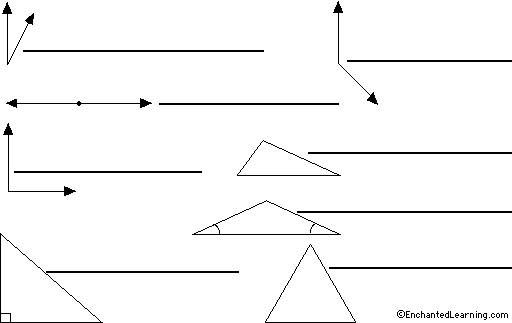EnchantedLearning.com is a user-supported site.
As a bonus, site members have access to a banner-ad-free version of the site, with print-friendly pages.

 You might also like: Going Up at a 40-Degree Angle Triangle Classification Worksheet Label Protractor Diagram Print-out Label Math Symbols Diagram Print-out Label the Quadrilaterals Printout Today's featured page: Cloze (Fill-in-the-Blank) - Astronomy

 AnswersMore on Triangles EnchantedLearning.comLabel Angles and Triangles More Label Me! printouts
Read the definitions, then label the angles and triangles below.
 acute angle - an angle that is less than 90°. equilateral triangle - a triangle whose sides are all the same length. isosceles triangle - a triangle that has two sides the same length (and two angles the same). obtuse angle - an angle that is greater than 90°. right angle - an angle that measures exactly 90°. right triangle - a triangle that has one interior angle that is exactly 90°. Note, some right triangles are also scalene triangles. scalene triangle - a triangle whose sides are all different lengths. Note, some scalene triangles are also right triangles. straight angle - an angle that measures 180°.## Enchanted Learning Search

 Search the Enchanted Learning website for: